# Removing Radicals from the Denominator

 For an answer to be in proper format, radicals (square roots) cannot be left in the denominator. The way to remove them from the denominator is to multiply both the numerator and the denominator by the radical. Here's how it works: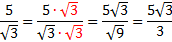We started by multiplying the numerator and denominator by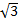. Then, we simplified the top and the bottom. Let's look at a couple more examples.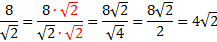We started by multiplying the numerator and denominator by. Then, we simplified the top and the bottom. At the last step, we reduced 8/2 to get 4. Note that you can only reduce numbers that are either both inside or both outside the square root.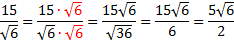Notice in the last step that the 6's will not reduce because one is inside the square root and one is outside. However, 15/6 will reduce to 5/2. Practice: Rewrite the expression without a radical in the denominator.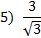Answers: 1)2)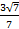3)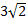4)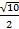5)